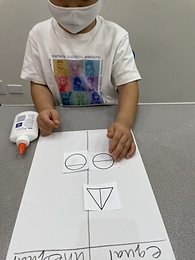## Ms. Kayla

### Target 1​

###### Lesson Type:

Continuation

Number Operation

:

Number System

Understand that numbers have an order (limited to numbers 0 to 50).

###### 1:

Understand that the number sequence does not change, even when some numbers are missing from a given sequence.

###### 2:

Identify when a given sequence is a correct count sequence.

###### 3:

Understand that the last number counted depicts the quantity of the objects.

Pre-K

###### Vocabulary:

Number, Order, Missing, Next

Activities:

!. Students were given and presented with number cards. Students were asked to put the cards in order. Students identified their numbers, and while they were counting, had to determine whose number would go next in the number sequence. Once the numbers were in order, the teacher took away one card in the sequence. Students had to identify which number was missing and where it would go in the count sequence.

2. Number cards 1-10 were laid out on the carpet. Students jumped from one number to the next in the correct number order. Students were also presented with the numbers 11-20 and continued in the same way, counting in number order.

3. Students were given a color and had to collect the fruits or vegetables of the same color. Students then counted out the number of frutis or vegetables they collected. Then they selected the number card that matched their number of fruits or vegetables.### Home Exploration

###### Guiding Questions:## Absent Students:

### Target 2

:

###### 1:

(This is an informal introduction to fractions.)

###### 2:

Distinguish between equal and unequal parts.

###### 3:

Describe if parts are the same or if one is bigger or smaller.

Pre-K

###### Vocabulary:

Equal, Unequal, Same, Different, Bigger, Smaller, Half

Activities:

1. Students used playdough and shapes to identify equal and unequal parts of a whole. The teacher showed the students, with playdough, what unequal meant - one part is bigger or smaller than the other. Students were introduced to the word unequal. Then students were shown, with playdough, what equal meant - two or more pieces of the same size/length. Students then practiced cutting their playdough into equal and unequal parts.

2. Students completed a chart for equal and unequal parts. Students looked at shapes that were divided into two parts. Students had to determine whether the parts were equal or not and put it in the correct column.

3. Students worked on a worksheet given two sets of objects. Students counted the objects in each set and had to say if they were equal or not.### Home Exploration

###### Guiding Questions:### Target 3

:

###### Vocabulary:

Activities:### Home Exploration